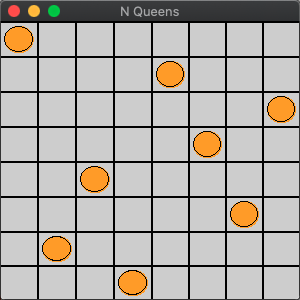# Eight queens puzzle

According to the wikipedia, the eight queens puzzle is the problem of placing eight chess queens on an 8×8 chessboard so that no two queens threaten each other; thus, a solution requires that no two queens share the same row, column, or diagonal.

One of the solutions is as follows,The eight queens or N queens problem is generally divided into finding all solutions or a particular solution.

## find all solutions

Here, we quote the algorithm from SICP Chapter 2, Exercise 2.42, described roughly as follows,

1. Suppose we have found all valid placements of the queens in the first N-1 columns, denoted S{P1, P2, …}

2. For each of the placements in S, we place the queens on each row of the Nth column, thus forming a new placement, denoted S’{P1R1, P1R2 … P1Rn, P2R1, … P2Rn, …}

3. From the new placement S’, all valid placements are filtered out, which is the final result.

The main Python code is as follows:

``````def n_queens(n):
"""return all the placements
"""
if n == 0:
# no placement if n is 0
return [[]]

# valid placement of the first N-1 columns
placements = n_queens(n-1)

new_placements = []
for row in range(n):
# adjoin each row of the Nth column to the previous placement to form a new placement

# finally filter out effective placement
return filter_out_valid(new_placements)
``````

In the above code, `adjoin` function will place the Nth column of queens adjacent to the beginning of each placement, so that later in the filter function, we only need to determine whether the first queen attacks with the rest queens .

The main Scheme code is as follows:

``````(define (queens board-size)
(define (queen-cols k)
(if (= k 0)
(list empty-board)
(filter
(lambda (positions) (safe? k positions))
(flatmap
(lambda (rest-of-queens)
(map (lambda (new-row)
(enumerate-interval 1 board-size)))
(queen-cols (- k 1))))))
(queen-cols board-size))
``````

Scheme code is written in declarative style, i.e. functional programming, using a combination of `filter`, `map`, which is more readable than the imperative style.

Full code：queens.py queens.ss

## find a particular solution

We quote the algorithm from HtDP 28.2, described roughly as follows,

1. Initialize the board, all squares are available for placement

2. Find the first place on the board where we can place the queen, and set the same row/column/diagonal to unplaceable

3. Find the remaining squares from the board that can be placed

1. If there are any, repeat step 2

2. If not, the position found in step 2 does not produce the final result and go back to the next available position in step 2

4. Repeat 2 until all N queens have been placed or there are no more places to place them

The main Python code is as follows:

``````# initialize a two-dimensional array as a blank board, with all values set to False
board = build_board(n)

def n_queens(n, board):
if n == 0:
# N queens are placed
return board

# find all available positions on the current board
positions = find_safe_positions(board)

for a_posn in positions:
new_board = placement(board, a_posn)
ret = n_queens(n-1, new_board)
if ret is False:
continue
else:
return ret
return False
``````

`placement` places the queen in a position on the board, while setting the position on the same row/column/diagonal to unplaceable, and returns a new board.

The main Scheme code is as follows:

``````;; placement : Board Nat -> board or false
;; places n queens on a-board. if possible, returns
;; the board. otherwise, returns false
(define (placement a-board n)
(cond
[(zero? n) a-board]
[else
(let [(possible-places (find-open-spots a-board))]
(placement/list possible-places a-board n)]))

;; placement/list : (Listof Posn) Board Nat -> board or false
;; tries to place n queens in all of the boards. As soon as
;; one of them works out, it returns that one. If none
;; work out, returns false.
(define (placement/list possible a-board n)
(cond
[(null? possible) false]
[else (let [(possible-posn (car possible))]
(c (placement new-board (sub1 n)))]
(cond
[(boolean? c)
(placement/list (cdr possible) a-board n)]
[else c])
))]))
``````

The Scheme code uses mutually recursive, which brings the code closer to the problem formulation.

Full code：queens.py queens.ss

Tags:

Categories:

Updated: